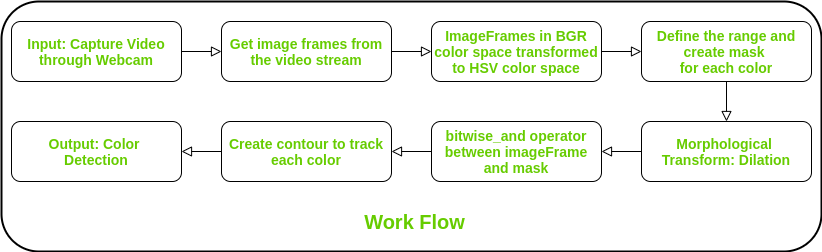Related Articles

# Multiple Color Detection in Real-Time using Python-OpenCV

• Last Updated : 10 May, 2020

For a robot to visualize the environment, along with the object detection, detection of its color in real-time is also very important.

### Why this is important? : Some Real-world Applications

• In self-driving car, to detect the traffic signals.
• Multiple color detection is used in some industrial robots, to performing pick-and-place task in separating different colored objects.

This is an implementation of detecting multiple colors (here, only red, green and blue colors have been considered) in real-time using Python programming language.
Python Libraries Used:

### Work Flow Description:

Step 1: Input: Capture video through webcam.
Step 2: Read the video stream in image frames.
Step 3: Convert the imageFrame in BGR(RGB color space represented as three matrices of red, green and blue with integer values from 0 to 255) to HSV(hue-saturation-value) color space. Hue describes a color in terms of saturation, represents the amount of gray color in that color and value describes the brightness or intensity of the color. This can be represented as three matrices in the range of 0-179, 0-255 and 0-255 respectively.
Step 4: Define the range of each color and create the corresponding mask.
Step 5: Morphological Transform: Dilation, to remove noises from the images.
Step 6: bitwise_and between the image frame and mask is performed to specificaly detect that particular color and discrad others.
Step 7: Create contour for the individual colors to display the detected colored region distinguishly.
Step 8: Output: Detection of the colors in real-time.Below is the implementation.

 `# Python code for Multiple Color Detection`` ` ` ` `import` `numpy as np``import` `cv2`` ` ` ` `# Capturing video through webcam``webcam ``=` `cv2.VideoCapture(``0``)`` ` `# Start a while loop``while``(``1``):``     ` `    ``# Reading the video from the``    ``# webcam in image frames``    ``_, imageFrame ``=` `webcam.read()`` ` `    ``# Convert the imageFrame in ``    ``# BGR(RGB color space) to ``    ``# HSV(hue-saturation-value)``    ``# color space``    ``hsvFrame ``=` `cv2.cvtColor(imageFrame, cv2.COLOR_BGR2HSV)`` ` `    ``# Set range for red color and ``    ``# define mask``    ``red_lower ``=` `np.array([``136``, ``87``, ``111``], np.uint8)``    ``red_upper ``=` `np.array([``180``, ``255``, ``255``], np.uint8)``    ``red_mask ``=` `cv2.inRange(hsvFrame, red_lower, red_upper)`` ` `    ``# Set range for green color and ``    ``# define mask``    ``green_lower ``=` `np.array([``25``, ``52``, ``72``], np.uint8)``    ``green_upper ``=` `np.array([``102``, ``255``, ``255``], np.uint8)``    ``green_mask ``=` `cv2.inRange(hsvFrame, green_lower, green_upper)`` ` `    ``# Set range for blue color and``    ``# define mask``    ``blue_lower ``=` `np.array([``94``, ``80``, ``2``], np.uint8)``    ``blue_upper ``=` `np.array([``120``, ``255``, ``255``], np.uint8)``    ``blue_mask ``=` `cv2.inRange(hsvFrame, blue_lower, blue_upper)``     ` `    ``# Morphological Transform, Dilation``    ``# for each color and bitwise_and operator``    ``# between imageFrame and mask determines``    ``# to detect only that particular color``    ``kernal ``=` `np.ones((``5``, ``5``), ``"uint8"``)``     ` `    ``# For red color``    ``red_mask ``=` `cv2.dilate(red_mask, kernal)``    ``res_red ``=` `cv2.bitwise_and(imageFrame, imageFrame, ``                              ``mask ``=` `red_mask)``     ` `    ``# For green color``    ``green_mask ``=` `cv2.dilate(green_mask, kernal)``    ``res_green ``=` `cv2.bitwise_and(imageFrame, imageFrame,``                                ``mask ``=` `green_mask)``     ` `    ``# For blue color``    ``blue_mask ``=` `cv2.dilate(blue_mask, kernal)``    ``res_blue ``=` `cv2.bitwise_and(imageFrame, imageFrame,``                               ``mask ``=` `blue_mask)``  ` `    ``# Creating contour to track red color``    ``contours, hierarchy ``=` `cv2.findContours(red_mask,``                                           ``cv2.RETR_TREE,``                                           ``cv2.CHAIN_APPROX_SIMPLE)``     ` `    ``for` `pic, contour ``in` `enumerate``(contours):``        ``area ``=` `cv2.contourArea(contour)``        ``if``(area > ``300``):``            ``x, y, w, h ``=` `cv2.boundingRect(contour)``            ``imageFrame ``=` `cv2.rectangle(imageFrame, (x, y), ``                                       ``(x ``+` `w, y ``+` `h), ``                                       ``(``0``, ``0``, ``255``), ``2``)``             ` `            ``cv2.putText(imageFrame, ``"Red Colour"``, (x, y),``                        ``cv2.FONT_HERSHEY_SIMPLEX, ``1.0``,``                        ``(``0``, ``0``, ``255``))    `` ` `    ``# Creating contour to track green color``    ``contours, hierarchy ``=` `cv2.findContours(green_mask,``                                           ``cv2.RETR_TREE,``                                           ``cv2.CHAIN_APPROX_SIMPLE)``     ` `    ``for` `pic, contour ``in` `enumerate``(contours):``        ``area ``=` `cv2.contourArea(contour)``        ``if``(area > ``300``):``            ``x, y, w, h ``=` `cv2.boundingRect(contour)``            ``imageFrame ``=` `cv2.rectangle(imageFrame, (x, y), ``                                       ``(x ``+` `w, y ``+` `h),``                                       ``(``0``, ``255``, ``0``), ``2``)``             ` `            ``cv2.putText(imageFrame, ``"Green Colour"``, (x, y),``                        ``cv2.FONT_HERSHEY_SIMPLEX, ``                        ``1.0``, (``0``, ``255``, ``0``))`` ` `    ``# Creating contour to track blue color``    ``contours, hierarchy ``=` `cv2.findContours(blue_mask,``                                           ``cv2.RETR_TREE,``                                           ``cv2.CHAIN_APPROX_SIMPLE)``    ``for` `pic, contour ``in` `enumerate``(contours):``        ``area ``=` `cv2.contourArea(contour)``        ``if``(area > ``300``):``            ``x, y, w, h ``=` `cv2.boundingRect(contour)``            ``imageFrame ``=` `cv2.rectangle(imageFrame, (x, y),``                                       ``(x ``+` `w, y ``+` `h),``                                       ``(``255``, ``0``, ``0``), ``2``)``             ` `            ``cv2.putText(imageFrame, ``"Blue Colour"``, (x, y),``                        ``cv2.FONT_HERSHEY_SIMPLEX,``                        ``1.0``, (``255``, ``0``, ``0``))``             ` `    ``# Program Termination``    ``cv2.imshow(``"Multiple Color Detection in Real-TIme"``, imageFrame)``    ``if` `cv2.waitKey(``10``) & ``0xFF` `=``=` `ord``(``'q'``):``        ``cap.release()``        ``cv2.destroyAllWindows()``        ``break`

Output:

Attention geek! Strengthen your foundations with the Python Programming Foundation Course and learn the basics.

To begin with, your interview preparations Enhance your Data Structures concepts with the Python DS Course. And to begin with your Machine Learning Journey, join the Machine Learning – Basic Level Course

My Personal Notes arrow_drop_up